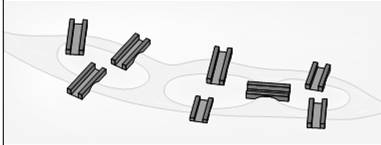# Recreation The illustration below depicts bridges connecting islands in a river to the banks. Can a person start at one location and take a stroll so that each bridge is crossed once but no bridge is crossed twice? (The person does not need to return to the starting location.) Draw a graph to represent the land areas and bridges. Answer the question using the graph to explain your reasoning.### Mathematical Excursions (MindTap C...

4th Edition
Richard N. Aufmann + 3 others
Publisher: Cengage Learning
ISBN: 9781305965584

#### Solutions

Chapter
Section### Mathematical Excursions (MindTap C...

4th Edition
Richard N. Aufmann + 3 others
Publisher: Cengage Learning
ISBN: 9781305965584
Chapter 5, Problem 4T
Textbook Problem
4 views

## Recreation The illustration below depicts bridges connecting islands in a river to the banks. Can a person start at one location and take a stroll so that each bridge is crossed once but no bridge is crossed twice? (The person does not need to return to the starting location.) Draw a graph to represent the land areas and bridges. Answer the question using the graph to explain your reasoning.To determine

To sketch:

The given geographical map as a graph and evaluate whether a person can start at a point and crosses the bridges exactly once and return to the same point.

### Explanation of Solution

Given:

Calculation:

The zonal situation of the given picture is represented as graph below.

The graph is given below

### Still sussing out bartleby?

Check out a sample textbook solution.

See a sample solution

#### The Solution to Your Study Problems

Bartleby provides explanations to thousands of textbook problems written by our experts, many with advanced degrees!

Get Started

Find more solutions based on key concepts
In problems 23-36, use the rules of exponents to simplify so that only positive exponents remain. 35.

Mathematical Applications for the Management, Life, and Social Sciences

Solve the equations in Exercises 126. x44x2=4

Finite Mathematics and Applied Calculus (MindTap Course List)

Find a formula for the inverse of the function. 26. y=1ex1+ex

Single Variable Calculus: Early Transcendentals, Volume I

Show that (nk)=(nnk). Give an interpretation involving subsets.

Probability and Statistics for Engineering and the Sciences

In Exercises 35-38, find an equation of the line that has slope m and y-intercept b. 37. m = 0; b = 5

Applied Calculus for the Managerial, Life, and Social Sciences: A Brief Approach

Factor each trinomial completely: a2+2a63

Elementary Technical Mathematics

Find the area of the shaded region.

Single Variable Calculus: Early Transcendentals

Sometimes, Always, or Never: If limxaf(x) and f(a) both exist, then f is continuous at a.

Study Guide for Stewart's Single Variable Calculus: Early Transcendentals, 8th

True or False: a × b is a vector in the plane formed by vectors a and b.

Study Guide for Stewart's Multivariable Calculus, 8th